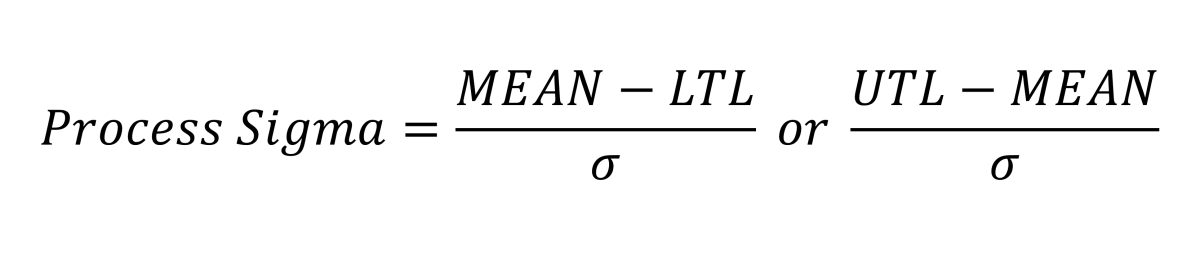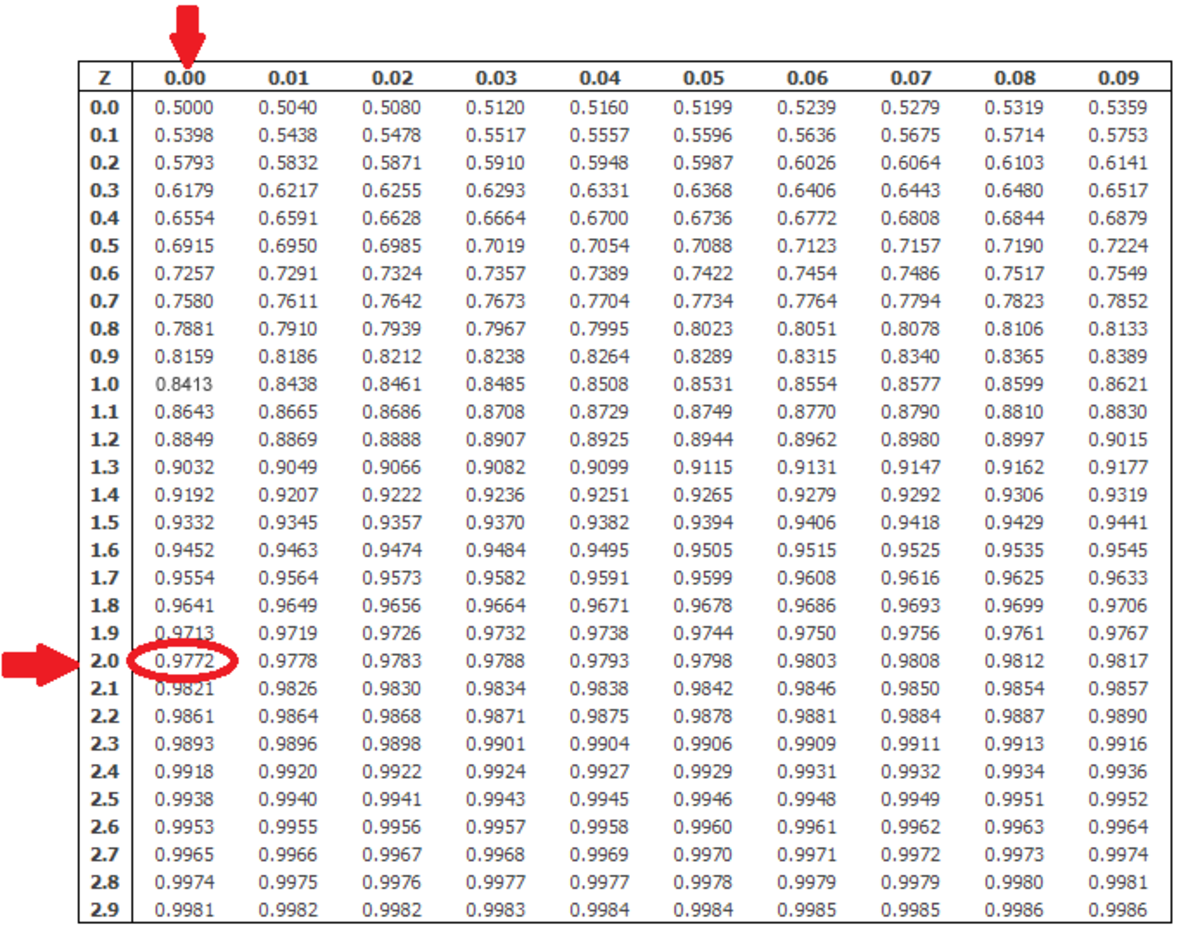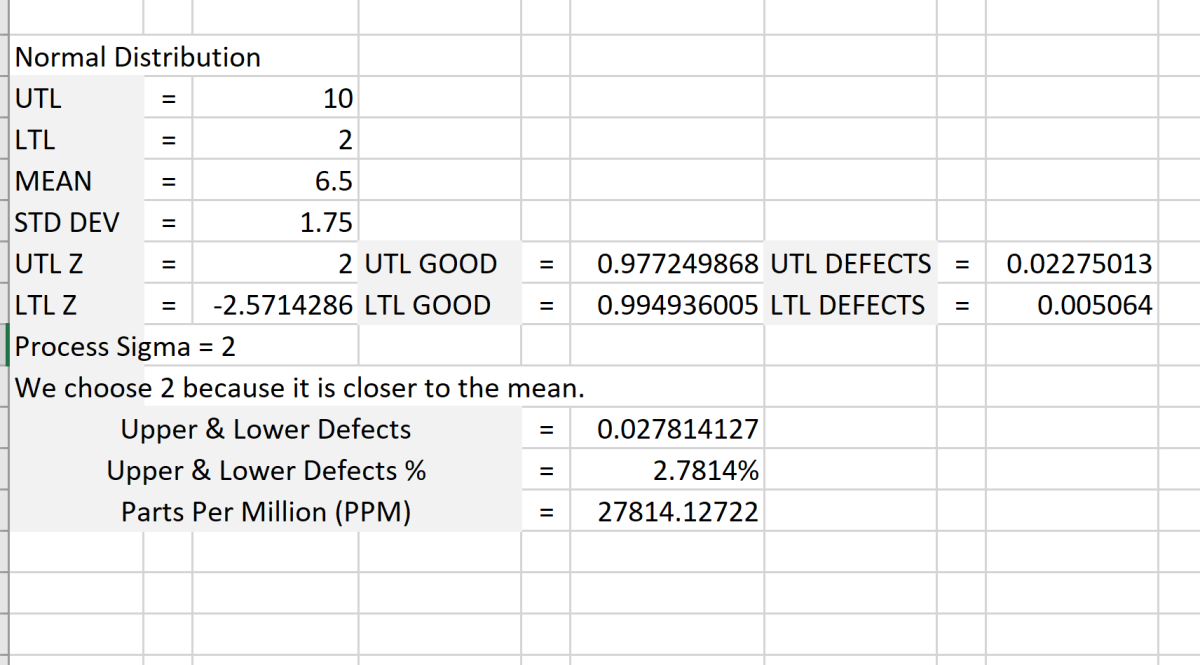# Calculating the Six Sigma Level of Processing

Joshua is a graduate student at the USF. He has interests in business technology, analytics, finance, and lean six sigma.Six Sigma was developed by an engineer named Bill Smith of the Motorola company in 1989. The system of techniques and tools that make up Six Sigma are becoming more widely used today in manufacturing to enhance quality and promote improvement. Created by Joshua Crowder

## What Is Process Sigma?

Process sigma is a measure of the variation in a process relative to requirements. Requirements are set as an upper tolerance limit (UTL) and a lower tolerance limit (LTL). The term process sigma is the same as the statistical z-score.

With an assumption that a process being measured falls under a normal distribution, the distance between the mean of the process and the UTL or LTL is the process sigma. So, the higher your process sigma is, the better your process is. The reason for this is that the distribution is narrowing at the mean as the process sigma increases.

Six Sigma is one of the best tools to keep processes from varying. You must have an acceptable quality range, the mean, and a calculated standard deviation (σ), which is used to quantify the amount of variation or dispersion of your data. With this data, the process sigma (z-score) can be calculated. To find this measure, either the UTL or the LTL can be used. The equations to find process sigma can be found below.The equations above are used to find process sigma. The lowest of the two outcomes will be called process sigma. Created by Joshua Crowder

## Example of Using the Process Sigma Equation

This example involves measurements for a part that needs to be between the range of 2 and 10 inches. The LTL will be 2 and the UTL will be 10. From past data collected on this process, the mean of the process is 6.5 and the standard deviation is 1.75. Let's first use the UTL to calculate the sigma level.

(10 - 6.5) /1.75 = 2.0

Process sigma for the UTL is 2.0

(6.5-2) /1.75 = 2.57

Process sigma for the LTL is 2.57

Process Sigma = 2

We choose 2 because 2 is closer to the mean than 2.57, giving us more defects. If we want to go a few steps further, we can calculate a few more items. To find the area of defective parts outside of the upper tolerance limit, we must take the process sigma (z-score) "2.0" and find 2.00 on a normal distribution chart.

So, as you can see on the chart below, to find the z-score you must find 2.0 on the horizontal axis then find .00 on the vertical axis. The vertical axis is for the hundredth place only. Find the z-scores in the chart below.The normal distribution table is used to find the percentage of the area from the mean to the process sigma (z-score).Created by Joshua Crowder

### Upper Tolerance Limit

Process sigma for the UTL is 2.0

Scroll to Continue

Probability of good outcome = .9772

Probability of a bad outcome 1- .9771 = .0229

### Lower Tolerance Limit

Process sigma for the LTL is 2.57

Probability of good outcome = .9949

Probability of a bad outcome 1-.9949 = .0051

After finding the z-scores on the chart, we can say when operating at a level above the level of the mean we have a 97.72 percent chance of having a good outcome with the UTL and a 99.49 chance of having a good outcome with LTL.

The probability of having defective products can be found simply by subtracting the probability of a good outcome from 1. Adding these probabilities together will give you the upper and lower defect percentage (.51 + .0229 = .028 or 2.8%). Now if you multiply the probability of having bad parts to 1 million, we can now say that this process has 2,800 defective parts per million opportunities (DPMO). See the visual representation below for the outcome percentages.

## Use of Microsoft Excel to Find Process Sigma

Microsoft Excel can be utilized to find process sigma using a series of steps. You can download the Microsoft Excel example here. As you can see in the picture below, all calculations can be made in Microsoft Excel.

These are the Microsoft Excel Statistical functions used to calculate process sigma:

=STANDARDIZE (Calculates process sigma)

=NORM.S.DIST (Left-tail defective percentage from negative z-score)

=NORM.S.DIST (UTL good parts percentage)

=NORM.S.DIST(ABS (UTL good parts percentage from the negative z-score)

=1-NORM.S.DIST (Right-tail Defective percentage)Process sigma calculations can be made in Microsoft Excel with the help of statistical functions. Created by Joshua Crowder

Boyer, K. & Verma, R. (2010). Operations & supply chain management for the 21st century. Mason, OH: South-Western.

This content is accurate and true to the best of the author’s knowledge and is not meant to substitute for formal and individualized advice from a qualified professional.